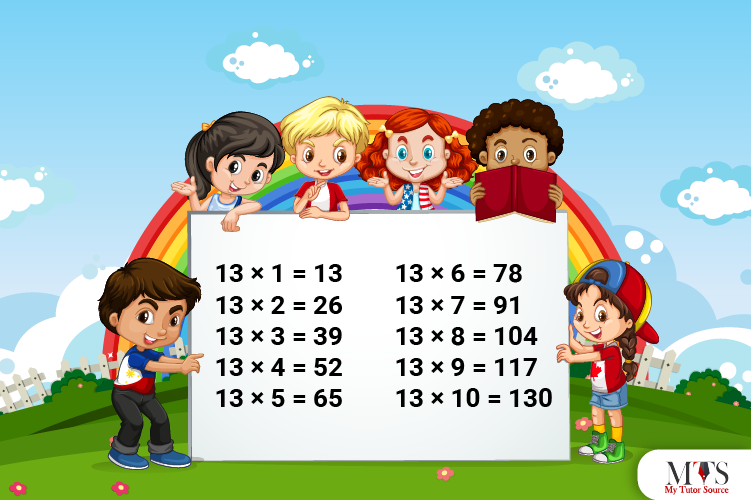# Multiplication Table of 13 – Tips to Memorize 13 Times Table & Example QuestionsTo enrich mathematical skills, memorizing multiplication tables is important. Few multiplication tables are hard to learn and memorize, such as the 13 times table. So let us know how to remember the 13 times table with tips. In this article, we have added a few solved example questions and a table of 13 for practice purposes. The daily use of multiplication tables is natural, and if a student needs extra help to hone their calculating skills, they must hire a private math tutor. They not only reinforce the importance of learning but also help in memorizing the multiplication tables.
Let us learn the table of 13!

## Table of 13

This section includes the complete 13 times table up to 20. It is recommended to memorize the table in parts as the results of the multipliers are large numbers.

13 x 1 = 13
13 x 2 = 26
13 x 3 = 39
13 x 4 = 52
13 x 5 = 65
13 x 6 = 78
13 x 7 = 91
13 x 8 = 104
13 x 9 = 117
13 x 10 = 130
13 x 11 = 143
13 x 12 = 156
13 x 13 = 169
13 x 14 = 182
13 x 15 = 195
13 x 16 = 208
13 x 17 = 221
13 x 18 = 234
13 x 19 = 247
13 x 20 = 260

## Method to Read Table of 13

The 13 times table or table of 13 can be written in words in the following way. However, to memorize the table, students must also read that in the given method.

Thirteen ones are thirteen (13)
Thirteen twos are twenty-six (26)
Thirteen threes are thirty-nine (39)
Thirteen fours are fifty-two (52)
Thirteen fives are sixty-five (65)
Thirteen sixes are seventy-eight (78)
Thirteen sevenths are ninety-one (91)
Thirteen eighths are one hundred and four (104)
Thirteen nines are one hundred and seventeen (117)
Thirteen tens are one hundred and thirty (130)
Thirteen elevens are one hundred and forty-three (143)
Thirteen twelves are one hundred and fifty-six (156)
Thirteen thirteenths are one hundred and sixty-nine (169)
Thirteen fourteens are one hundred and eighty-two (182)
Thirteen fifteenths are one hundred and ninety-five (195)
Thirteen sixteenths are two hundred and eight (208)
Thirteen seventeens are two hundred and twenty-one (221)
Thirteen eighteenths are two hundred and thirty-four (234)
Thirteen nineteens are two hundred and forty-seven (247)
Thirteen twenties are two hundred and sixty (260)

## Tips for Memorizing Multiplication Table of 13

Some students have a hard time learning and memorizing multiplication tables at school. Here are the most straightforward tips for struggling students to learn 13 times table fast:

• Recall 3 times table and add natural numbers (multipliers) to the tens’ digit of its product to generate the results of 13 times table.
• Another tip for memorizing a table of 13 is adding 13 into the prior results to get the results of the next multipliers. For example, like 13 x 3 = 39, and to calculate the 13 x 4, add 13 into 39, which is 52. Thus, 13 x 4 = 52

### Example Question of Table of 13

Question: Find a number before 13 times 9 and after 13 times 4.

Solution:

Given that,
13 times 9 = 13 x 9 = 117
Number before 13 times 9 = 117 – 1 = 116
Also,
13 times 4 = 13 x 4 = 52
Number after 13 times 4 = 52 + 1 = 53
Hence, the number before 13 times 9 and 13 times 4 is 116 and 53, respectively.

Question: Find 16 less than 13 times 3.

Solution:

Given that,
16 less than 13 times 3
In mathematical form,
= 13 x 3 – 16
= 39 – 16
= 23
Hence, 16 less than 13 times 3 is 23

Question: What is 6 multiplied by 13?

Solution:

Using the table of 13
⇒ 6 x 13 = 78
Hence, 6 multiplied by 13 is 78

Question: If 13 students are in a group and there are a total of 9 groups, then how many students are there? Solve using the multiplication table.

Solution:

Number of students = 13
Total groups = 9
Using the table of 13,
⇒ 13 x 9 = 117
Hence, there are a total of 117 students.

Question: Using the table of 13, find the value of 13 times 5 plus 13.

Solution:
Given that,
13 times 5 plus 13
In mathematical form,
= 13 x 5 + 13
= 65 + 13
= 78
Hence, 13 times 5 plus 13 is 78.

Question: What is the cube of thirteen?

Solution:

In mathematical form, the cube of thirteen is written as,
⇒ 133 = 13 x 13 x 13 = 2197
Thus, the cube of thirteen is 2197.

### Find Top Tutors in Your AreaWith over 3 years of experience in teaching, Chloe is very deeply connected with the topics that talk about the educational and general aspects of a student's life. Her writing has been very helpful for students to gain a better understanding of their academics and personal well-being. I’m also open to any suggestions that you might have! Please reach out to me at chloedaniel402 [at] gmail.com